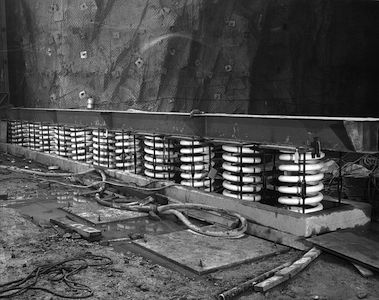# The local time is 3 o'clockThere are some things in physics that we are so used to taking for granted that we don't even think about them. Consider, for example, the statement that classical physics is local - physical systems involve interactions that occur at the same points in space and at the same times. Does this have to be so? Let's look at a simple spring to see what happens to physics if we make things a little non-local.

The equation of motion for the position of a mass $m$ at the end of a spring with spring constant $k$ is

$\frac{d^2 x(t)}{dt^2}=-\frac{k}{m} x(t)$

if the other end of the spring is fixed in space. Consider solutions to this equation of the form $x = e^{iEt}$ where $E$ is a constant (which is a priori possibly complex). Let $N$ be the number of possible values for $E$ that satisfy this equation.

We'll now make the spring equation slightly non-local in time. We do this by letting the acceleration of the mass be determined by not just $x(t)$, but by a combination of the position at two different times, $x(t-\Delta t)$ and $x(t+\Delta t)$, where $\Delta t$ is a constant. The new equation of motion is

$\frac{d^2 x(t)}{dt^2}=-\frac{k}{2m}( x(t+\Delta t) + x(t-\Delta t))$

so that as $\Delta t \rightarrow 0$ we return to the usual equation for a spring. This second equation with $\Delta t \neq 0$ also has solutions of the form $x=e^{i E t}$ for certain values of $E$. Let $M$ be the number of possible values of $E$ that satisfy this second equation of motion. What is $N/M$?

Hint: don't make any extra assumptions about $E$ than you absolutely need to.

×